# Module 6: Radiation Detection and Measurements

### 6.1 Introduction

Radiation cannot be perceived by human senses. There are two basic types of instruments used for its detection:

1. Particle counting instruments
2. Dose measuring instruments

The particle counting instruments are measuring the number of particles (electrons, alphas, protons, neutrons, etc.) or photons that give a signal in the detector, and give the result in counts per minute (cpm) or counts per second (cps). The dose-measuring instruments are also measuring the number of particles or photons, but the result is given in units of dose (R, Gy, Sv, etc.), or dose rate (R/s, mSv/h, Gy/min, etc.). Sometimes the same instrument is capable of both types of readings.

The internal dose can be estimated from bioassay measurements performed with particle counting instruments.

### 6.2 Particle counting instruments

Particle counting instruments are used to determine the activity of a sample taken from the environment, to measure the activity of body fluids, and can be used as portable survey instruments for contamination monitoring.

The detector in a particle counting instruments can either be a gas, a solid or liquid. When ionizing particles pass through the detector energy dissipation through a burst of ionization occurs. This burst of ionization is converted into an electrical pulse that actuates a readout device, such as a scaler or a ratemeter, to register a count.

#### 6.2.1 Gas-Filled Detectors

The gas-filled detectors resemble a cylindrical condenser, with a central anode for collecting electrons and an outer cathode for collecting positive ions. The ionizing particle passes through the gas that fills the condenser, creating positive ions and electrons.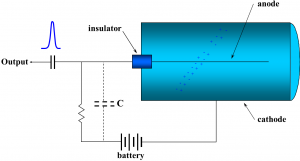There are various types of particle counting instruments filled with gas:

1. Ionization chamber counters (no secondary ions are produced)
2. Proportional counters (secondary ions are produced but the number is proportional to the initial energy of the radiation)
3. Geiger-Müller counters (secondary ions are produced in large numbers and the number of ions is no longer proportional with the radiation energy)

The main difference between these 3 types of counters simply depends on the voltage used for charging the condenser.

#### 6.2.2 Solid and Liquid Scintillation Detectors

A scintillation counter is a transducer that changes the kinetic energy of an ionizing particle into a flash of light. The flashes of light are viewed electronically by a photomultiplier tube. The output pulses may be amplified, sorted by size, and counted.

Different scintillation materials [NaI (Tl), CsI (Tl) crystals, plastics, or liquids] are used to detect different types of radiation.

Scintillation counters are widely used in our radiation protection program for bioassays, swipes, and laboratory experiment samples. Liquid scintillation counters have a very good detection threshold since the scintillation liquid and sample are practically mixed together.

When using a particle counting instrument, one must remember to measure a background first. The net number of counts is then obtained by subtracting the background from the actual number of counts produced by the source. Due to statistical processes for both nuclear disintegration and measurement itself, subtraction of the background is trivial (simple) only when the counting rates are much greater than the background. When they are close to each other, a statistical decision must be taken using the “detection limit” formula (DL):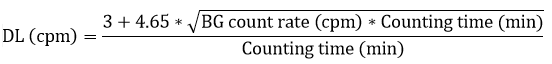Where “BG” means the background and “cpm” means “counts per minute”.

When the difference between sample measurement and background (net counting rates) is bigger than the detection limit, the measurement is considered statistically significant and the result is expressed as: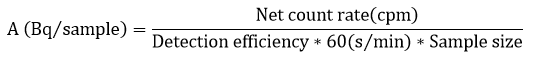The detection efficiency is determined by measuring samples of known activity (calibrated sources) under similar conditions. That is why it’s very important to have the detection system properly calibrated.

If a swipe sample is measured, the detection efficiency includes two terms:

1. Efficiency of the detector
2. Efficiency of the swiping process (to be on the conservative side at U of T, we consider a swiping efficiency of 10%)

The uncertainty of measurement is calculated with the formula: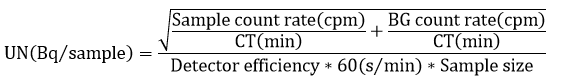The final result should be expressed as activity (± uncertainty), in Bq.

When the activity is expressed as Bq per volume of sample or per cm2, as is the case with the swipes, the volume of the sample (or the swiping surface) must also be accounted for in the denominator.

When the difference between sample measurement and background (net counts) is smaller than DL, the result of the measurement is NOT considered statistically significant and the result should be expressed as a minimum detectable activity (MDA).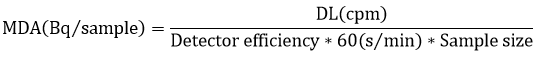MDA is not a characteristic of the sample measured but is a characteristic of the instrument’s limit for detecting radioactivity. That is why results should be expressed as “less than MDA (Bq)”. Such a result indicates that the radioactivity of the sample is less than the capability of the instrument for detecting radioactivity.

Nevertheless, this kind of result can be quite informative. It will indicate whether an instrument is appropriate for specific applications. For example, an instrument’s MDA might be equal to or greater than the release criteria for contaminated surfaces. Thus, any source whose activity is detected by the instrument cannot be released.

Example:
A tritium contamination occurred in a laboratory. A swipe of 100 cm2 was taken and measured with a liquid scintillation counter for 1 minute.

a) Considering a swipe efficiency of 10% and a detection efficiency of 55%. Determine the result of a measurement when the number of counts for the sample is 750 with a background of 41.

b) Decide to clean the area and swipe again. In the same conditions, determine the result of the measurement if the new reading is now 60 counts and the new background is 39.

a) The detection limit DL is: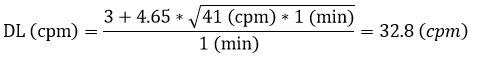The difference between the sample (750 cpm) and the background (41 cpm) is 709 cpm, which is more than 32.8 cpm. Therefore, the result is statistically significant. The activity and the uncertainty can now be calculated as: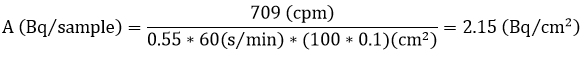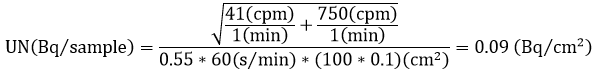Therefore the result is (2.15 ± 0.09) Bq/cm2

b) The new detection limit is: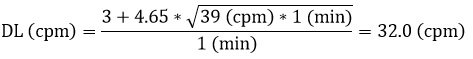The difference between the new sample (60 cpm) and the background (39 cpm) is now 21 cpm. Because 21 is smaller than 32, the measurement is not statistically significant. In this case, the MDA is: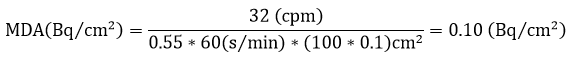Therefore, the result is expressed as “less than 0.10 Bq/cm2” (< 0.10 Bq/cm2) and indicates that the swipe sample is not contaminated.

### 6.3 Dose measuring instruments

To measure the radiation dose, the instrument’s response must be proportional to the energy absorbed from radiation. A “radiation flux” measuring instrument does not necessarily measure the dose. To effectively measure dose, the efficiency for different types of radiation and energy must be taken into account. Pocket dosimeters, film badges, and personal thermoluminescent dosimeters are all used to measure the personal dose. They are based on the effects of accumulated irradiation in the detector. Changes in the detector’s material can be stored for later reading of the results.

Thermoluminescent dosimeters (TLD) are used at U of T for measuring dose received by people working with radiation. A certified (by the federal regulatory agency) company provides this service to the university. There are two kinds of TLD used in U of T:

1. Whole-body dosimeter
1. This dosimeter should be worn somewhere on the person’s trunk.
2. Extremity dosimeter (a ring dosimeter)
1. This special dosimeter should be worn on the hand that is used the most during radioactive work.

Area dosimeters are identical with whole-body dosimeters and are used to measure the exposure in the area around the workplace.

All TLD dosimeters (whole-body, ring or area dosimeters) are limited to measuring high energy beta radiation and gamma/x-ray exposure. These dosimeters cannot measure exposure received from low-level energy beta emitters like H-3, C-14, S-35 or Ni-63.

Electronic dosimeters are also used for direct reading of dose. They are very useful for work in high radiation fields because of the alarm system they are equipped with. Alarms can be set for total dose, dose rate, and for superficial or deep dose.

Survey meters are particle counting instruments that have been calibrated to measure the dose. They are highly specialized and can only be used for the type of radiation (X-ray, gamma-ray or neutrons) for which they have been calibrated. These instruments should never be used to measure dose outside the energy range or type of radiation for which they were calibrated. The calibration of these instruments must be done according to a procedure approved by the federal regulator.

### 6.4 Internal dosimetry

As was mentioned before, inhalation and ingestion are the main paths for internal irradiation of those working with radioactive materials. Therefore, special methods are required for measuring the internal irradiation of personnel. At U of T, two internal dosimetry programs have been developed.

#### 6.4.1 Iodine measurement

The Iodine bioassay program is based on the well-known fact that iodine radionuclides used in our laboratories (I-125 and I-131) tend to accumulate inside the thyroid. Both radionuclides are gamma emitters. Therefore, a gamma detector can be used to measure the iodine content of the person’s thyroid. Proper calibration of the instrument is done using ‘phantoms’ that mimic human body composition. After gathering information about thyroid activity (in Bq) and the moment of iodine usage, we can estimate iodine uptake and intake. Since the level of radioiodine in the thyroid decreases after 5 days, the measurement must be done between 1 and 4 days after usage. The amount of radioiodine in the thyroid is compared with the annual limit on intake (ALI) and the dose received by the contaminated person can be estimated.

#### 6.4.2 Urinalysis

The scientific basis for this type of analysis is the fact that most of the radionuclides tend to be eliminated in body fluids. By measuring activity content in urine, we can estimate the uptake and the intake. The dose is estimated by comparing the intake with the ALI for that particular radionuclide.

Example:
a) During an iodine bioassay of a person using I-125, the result of a 2 minute measurement is 1200 counts (or 600 cpm). The background for a 2 minute measurement performed immediately before was 950 counts (or 475 cpm). Knowing the detection efficiency of the instrument for I-125 to be 1.42 % for a person, determine the content of I-125 in the person’s thyroid.

b) What is the content of I-125 for a different person measured immediately after the first one, if the measured value is now 980 counts (or 490 cpm)?

a) The detection limit for a 475 (cpm) background measurement is: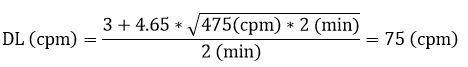The difference between the person’s measurement (600 cpm) and the background (475 cpm) is 125 cpm. This is more than 75 cpm. Therefore, the measurement is statistically significant. The activity of I-125 in the thyroid is:The uncertainty is:The result is: (147 ± 27) Bq

b) The second measurement is taken immediately after the first one. The assumption is made that the background of the instrument remains the same. Therefore, the detection limit is the same DL = 150 counts for a 2 minute measurement.

The difference between the person’s measurement (490 cpm) and background (475 cpm) is now 15 cpm. This number is less than 75 cpm. Therefore, the result is NOT statistically significant. The MDA of the instrument is: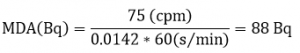The result is expressed as “less than 88Bq” (< 88 Bq), and the person’s thyroid is ‘clean’.